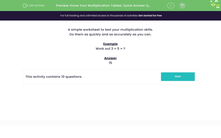# Multiply two 1 digit numbers

In this worksheet, students complete ten simple multiplications as quickly as possible using their tables knowledge.Key stage:  KS 2

Curriculum topic:   Number: Multiplication and Division

Curriculum subtopic:   Solve Multiplication/Division Problems

Popular topics:   Multiplication worksheets

Difficulty level:#### Worksheet Overview

In this activity, you will be using your multiplication skills.

Knowing your times tables facts by heart will be really useful to complete these questions.

Remember that multiplication is commutative. This means we can multiply numbers in any order and get the same product.

The product is the number we get when we multiply two numbers together.

Let's take a look at how this works.

If we wanted to multiply 6 x 5, there are two possible calculations.

6 × 5 = 30

5 × 6 = 30

Either way, the product is still 30.

Let's try an example question together.

Example

Work out 3 × 5

To work this out, we could either do 3 × 5 or 5 × 3.

3 × 5 = 15

5 × 3 = 15

Now it's your turn to try some questions like this.

### What is EdPlace?

We're your National Curriculum aligned online education content provider helping each child succeed in English, maths and science from year 1 to GCSE. With an EdPlace account you’ll be able to track and measure progress, helping each child achieve their best. We build confidence and attainment by personalising each child’s learning at a level that suits them.

Get started